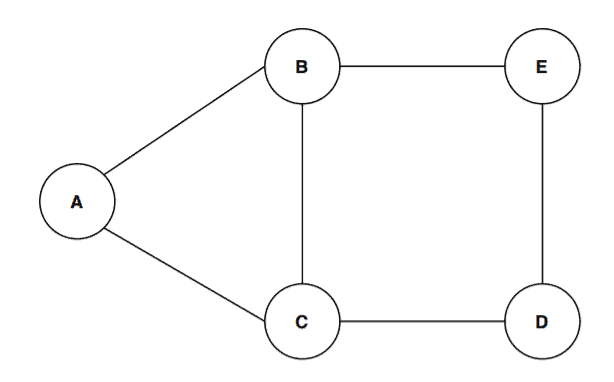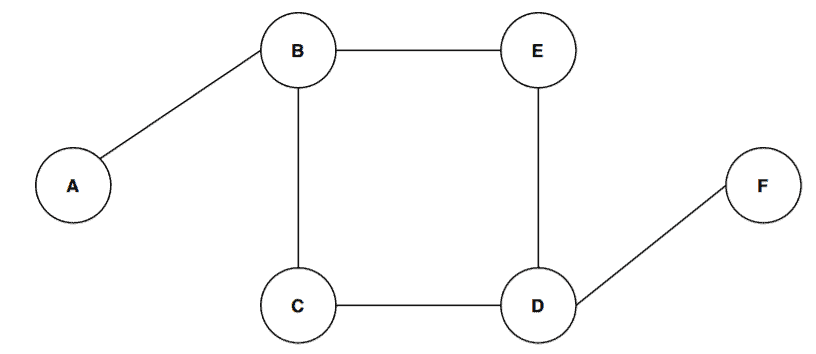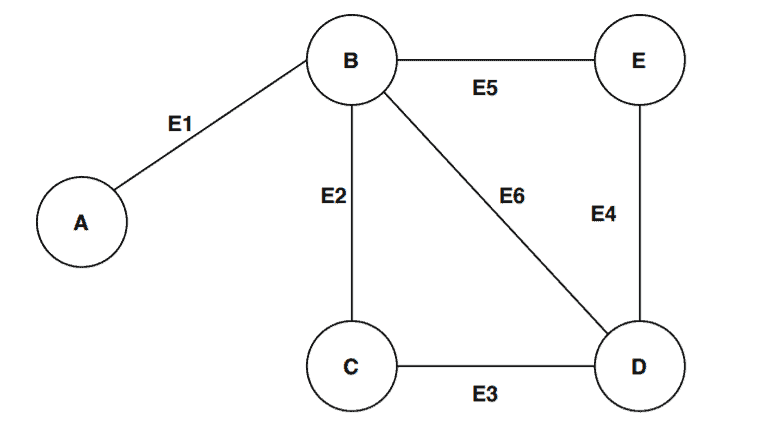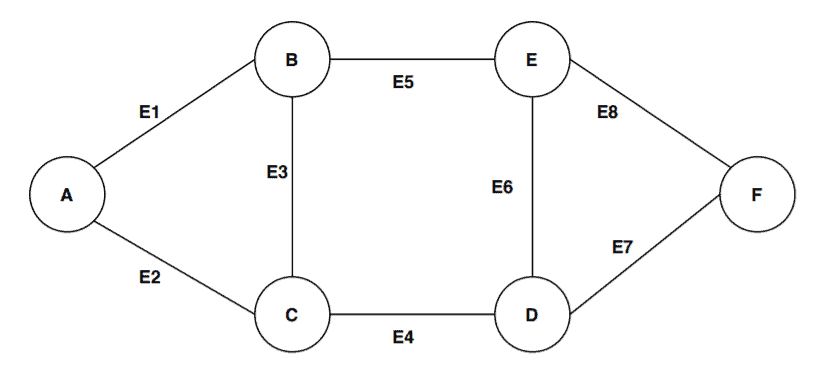## 1. Overview

In this article, we’ll discuss two common concepts in graph theory: Hamiltonian and Euler paths. We’ll start by presenting the general definition of both concepts and by showing some examples. We’ll also list some important properties of each concept.

Finally, we’ll present a high-level difference between the two concepts.

## 2. Definitions

Both Hamiltonian and Euler paths are used in graph theory for finding a path between two vertices. Let’s see how they differ.

### 2.1. Hamiltonian Path

A Hamiltonian path is a path that visits each vertex of the graph exactly once. A Hamiltonian path can exist both in a directed and undirected graph.

It’s important to discuss the definition of a path in this scope: It’s a sequence of edges and vertices in which all the vertices are distinct. The sequence of edges in a path can be finite as well as infinite.

So, if a path covers all the vertices of a given graph without repeating any vertex, then it’s a Hamiltonian path.

### 2.2. Euler Path

An Euler path is a walk where we must visit each edge only once, but we can revisit vertices. An Euler path can be found in a directed as well as in an undirected graph.

Let’s discuss the definition of a walk to complete the definition of the Euler path. A walk simply consists of a sequence of vertices and edges. Each vertex and edge can appear more than once in a walk. An Euler path restricts the walk by limiting each edge to appearing once.

So in short, if a walk covers all the edges of the graph exactly once, it is an Euler path.

## 3. Examples

### 3.1. Hamiltonian Path Example

Let’s take some graphs and try to find out if they’ve got any Hamiltonian paths.First, we’ll try empirically by taking a random path inabove, say. But, is it Hamiltonian?

Following the definition of a Hamiltonian path, our pathcovers all the vertices of our graph without visiting any vertex more than once. Hence we can say that the pathis a Hamiltonian path. Another example of a Hamiltonian path in this graph is.

Let’s take another graph and call it:Let’s try our method again and take the random path. Is it Hamiltonian?

According to the definition, a path shouldn’t contain a vertex more than once. Here we can see the vertexis repeated twice. So the pathis not a Hamiltonian path. In fact, there can’t be any Hamiltonian path for this graph.

### 3.2. Euler Path Example

Now, let’s try and do the same for Euler paths.Let’s define a walk in the graph. Our randomly picked sample walk inis. Is it follows the Eluer path definition? Let’s find out.

The walkcovers the edges. Now let’s see if our work satisfies the definition of an Euler path or not. As per the definition of an Euler path, a walk should cover all the edges without repeating any edge more than once. We can see our sample walk covers all the edges of the graphwithout repeating any edges.

Therefore we can conclude that the walk ABCDBED is an Euler path. Another example of an Euler path inis.

It’s time to consider a different graph:Again, we’ll follow the same procedure. We’re picking a random walkof the graph. Let’s investigate whether our picked walk satisfies the Euker path definition.

The edges it covers are. The walk covers all the edges of the graphbut there is repetition of the edgesandwhich is against the definition of an Euler path.

Therefore, we can conclude that the walkis not an Euler path. If we analyze the graphin detail, we’ll observe the graphcan’t contain any Euler path. It is not possible to cover all the edges without repeating vertices in.

## 4. Properties

There are some interesting properties associated with Hamiltonian and Euler paths. Let’s explore them.

### 4.1. Hamiltonian Path Properties

A simple graph withvertices contains a Hamiltonian path if

• The graph contains a pair of distinct non-adjacent vertices
• For each such pair, (degrees of vertex pair)(length of the shortest path for that pair)For a connected graph, a random pair of a distinct vertex is. Now according to the property :.

Here,,denotes the degree of the vertex,anddenotes the number of the vertex in. The other notationis used to denote the shortest path betweenandin.

Let’s verify this property with the graph. by picking two distinct non-adjacent vertices A and D:.

This is greater than the total number of vertex here in. Thus this property holds for.

In the case of a Hamiltonian path, it always starts and ends in different vertices. This property can be derived from the definition. In graph, we showed that the pathis a Hamiltonian path. It starts from the vertexand ends in. Hence, it follows the property.

### 4.2. Properties of Euler Path

If a graph has an Euler path, then the graph should have most two vertices with odd degrees.

In graph, the odd degree vertices areandwith degreeand. All other vertices are of even degree. Therefore, graphhas an Euler path.

On the other hand, the graphhas four odd degree vertices:. Therefore, the graphcan’t have an Euler path.

All the non-zero vertices in a graph that has an Euler must belong to a single connected component

## 5. A High-Level Comparison

In this section, we’re going to summarise all the theory that we discussed regarding Hamiltonian and Euler path and let’s present in an organized table format:## 6. Conclusion

In this article, we discussed Hamiltonian and Euler path in depth. We’ve presented a general definition followed by some examples to ensure the reader gets the fundamentals. Finally, we’ve gathered all the points we discussed and presented a high-level comparison between the two concepts.